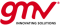If you wish to contribute or participate in the discussions about articles you are invited to contact the Editor

# DGNSS FundamentalsFundamentals
Title DGNSS Fundamentals
Edited by GMV
Level Basic
Year of Publication 2011The classical DGNSS technique is an enhancement to a primary GNSS system that consists of the determination of the GNSS position for an accurately-surveyed position known as reference station. DGNSS accuracy is in the order of 1 m (1 sigma) for users in the range of few tens of km from the reference station.

## The classical DGNSS technique

The standard DGNSS technique consists of the determination of the GNSS position from an accurately-surveyed position known as reference station. The method takes advantage of the slow variation with time and user position of the errors due to ephemeris prediction, residual satellite clocks, ionospheric and tropospheric delays. Starting from the reference station, the system computes and broadcasts either corrections to the GNSS position or to the pseudorange measurements to the DGNSS users. In order to be able to apply these corrections, the receiver has to be enabled for DGNSS and stay in the vicinity of the reference station to ensure that the two receivers (station and rover) observe the same GNSS satellite. Other uncorrelated errors (e.g. multipath) cannot be corrected by this method and specific techniques have to be applied to mitigate them.

DGNSS accuracy is in the order of 1 m (1 sigma) for users in the range of few tens of kilometers from the reference station, growing at the rate of 1 m per 150 km of separation. The United States Federal Radionavigation Plan and the IALA Recommendation on the Performance and Monitoring of DGNSS Services in the Band 283.5–325 kHz cite the United States Department of Transportation's 1993 estimated error growth of 0.67 m per 100 km from the broadcast site but measurements of accuracy across the Atlantic, in Portugal suggest a degradation of just 0.22 m per 100 km.

Variations of the method using corrections from multiple reference stations exist, leading to higher levels of accuracy.

## DGNSS Algorithm

The classical DGNSS algorithm is based on single differences of pseudorange observables. At a given epoch, and for a given satellite, the simplified pseudorange observation equation is the following:

$\displaystyle{ \qquad P =\rho+I+Tr+c(b_{Rx}-b_{Sat} )+\varepsilon_P \qquad \mbox{(1)} }$

Where:

$\displaystyle{ I }$ is the signal path delay due to the ionosphere;

$\displaystyle{ Tr }$ is the signal path delay due to the troposphere;

$\displaystyle{ b_{Rx} }$ is the receiver clock offset from the reference (GPS) time;

$\displaystyle{ b_{Sat} }$ is the satellite clock offset from the reference (GPS) time;

$\displaystyle{ c }$ is the vacuum speed of light;

$\displaystyle{ \varepsilon_P }$ are the measurement noise components, including multipath and other effects;

$\displaystyle{ \rho }$ is the geometrical range between the satellite and the receiver, computed as a function of the satellite $\displaystyle{ (x_{Sat}, y_{Sat},z_{Sat}) }$ and receiver $\displaystyle{ (x_{Rx}, y_{Rx},z_{Rx}) }$ coordinates as:

$\displaystyle{ \qquad \rho=\sqrt{〖(x_{Sat}-x_{Rx})〗^2+〖(y_{Sat}-y_{Rx})〗^2+〖(z_{Sat}-z_{Rx})〗^2 } \qquad \mbox{(2)} }$.

The next step is using a reference station at an accurately calibrated location $\displaystyle{ (x_o, y_o,z_o) }$, the reference-to-satellite range can be calculated as:

$\displaystyle{ \qquad R_o=\sqrt{〖(x_{Sat}-x_o)〗^2+〖(y_{Sat}-y_o)〗^2+〖(z_{Sat}-z_0)〗^2 } \qquad \mbox{(3)} }$.

When single differencing of both pseudoranges, $\displaystyle{ \Delta P = P_o - P }$, the ionospheric and tropospheric delays cancell out and also the satellite clock offset. The basic equation per satellite to solve is:

$\displaystyle{ \qquad \Delta P=\mathbf{{u}_o^{sat} } \Delta \mathbf{{x}} +c \Delta b_{Rx} +\Delta \varepsilon \qquad \mbox{(4)} }$

where $\displaystyle{ \mathbf{{u}_o^{sat} } }$ is the unitary vector between the reference station and the satellite. As there are not only one satellite in view, the estimation process is in fact a linear equation system, easily to solve. Depending on the distance between the rover and the reference stations, it could be needed to perform an iterative computation.